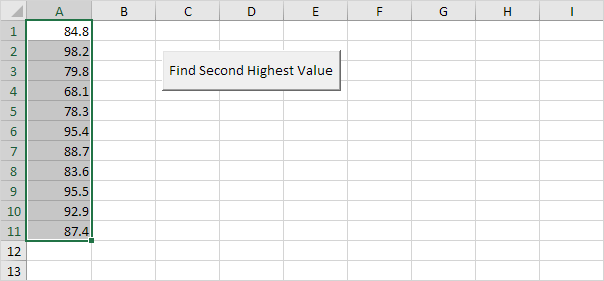# Find Second Highest Value

Below we will look at a program in Excel VBA that finds the second highest value.

Situation:1. First, we declare two Range objects and two variables of type Double. We call the Range objects rng and cell. One double variable we call highestValue, and one double variable we call secondHighestValue.

Dim rng As Range, cell As Range
Dim highestValue As Double, secondHighestValue As Double

2. We initialize the Range object rng with the selected range and the two Double variables with value 0.

Set rng = Selection
highestValue = 0
secondHighestValue = 0

3. First, we want to find the highest value. We want to check each cell in a randomly selected range (this range can be of any size). In Excel VBA, you can use the For Each Next loop for this. Add the following code lines:

'Find Highest Value
For Each cell In rng

Next cell

Note: rng and cell are randomly chosen here, you can use any names. Remember to refer to these names in the rest of your code. The green line is a comment and is only added here to provide information about this piece of code.

4. We check each cell in this range. If it's higher than highestValue, we write the value to the variable highestValue. Add the following code line to the loop.

If cell.Value > highestValue Then highestValue = cell.Value

Note: the first value is always higher than highestValue because the starting value of highestValue is 0.

5. Second, we want to find the second highest Value. We add another For Each Next loop.

'Find Second Highest Value
For Each cell In rng

Next cell

6. We check each cell in the selected range again. If it's higher than secondHighestValue and lower than highestValue, we write the value to the variable secondHighestValue. Add the following code line to the loop.

If cell.Value > secondHighestValue And cell.Value < highestValue Then secondHighestValue = cell.Value

7. Finally, we display the second highest value using a MsgBox.

MsgBox "Second Highest Value is " & secondHighestValue

8. Place your macro in a command button, select the numbers, and click on the command button.

Result:Note: understanding If Then statements and loops in Excel VBA is important if you want to write more complicated programs in the future. However, in this example, you can also use WorksheetFunction.Large to quickly find the second highest value.

9. The following code line produces the exact same result:

MsgBox "Second Highest Value is " & WorksheetFunction.Large(Range("A1:A11"), 2)

Note: use the value 3 to find the third highest value, the value 4 to find the fourth highest value, etc.

Go to Next Chapter: Loop